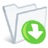SkinCalc 3.5.9
SkinCalc, which is completely free, features standard and advanced calculator functionalityKaren's Calculator
Karen's Calculator is a high-precision calculatorMetalogic Calculator
This calculator allows natural entry of equationCalculator Prompter
Calculator Prompter is a math expression calculatorEqualizer 1.77
Equalizer is a freeware scientific expression calculatorRedCrab 7.12.0
The calculator with fullscreen editorGeoGebra 6.0.355.0
Dynamic mathematics software used for geometry, algebra and calculusxFunc 3.3.0
Build complex math expressionsWord Count Mini 3.1.0
Word Count Mini is a tool to count Words, Lines, Pages and Characters.Precise Calculator 2.6
High precision scientific calculator for Windows.Calculator for Kids 1.0.0.0
A simple and lightweight application developed specifically for children, to provide them with a useful tool in learning basic mathsMeasurement Conversions for Windows 8 1.0.0.5
A lightweight, yet efficient application designed to convert between units such as mass, distance, volume, temperature, time, etcBitMaker 1.1.0
Convert decimal to binary with easeTk-Yupana Re 0.5.4
Tcl-based Incan Abacus emulatorLincalc 1.1.4
Powerful and easy-to-use calculatorTTCalc 0.9.3
TTCalc is an open source bignum mathematical calculator.12Ghosts Lookup 9.70
zip and area codes, acronyms, unit converter1st Calculator 1.15
Expression calculator and converter with skins and lots of features